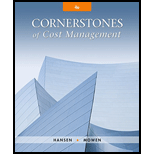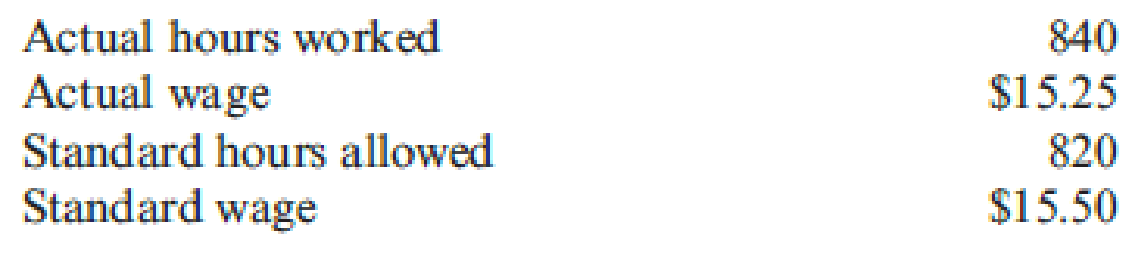# Relevant information for direct labor is as follows: What was the direct labor efficiency variance? a. $305 favorable b.$305 unfavorable c. $310 favorable d.$310 unfavorable### Cornerstones of Cost Management (C...

4th Edition
Don R. Hansen + 1 other
Publisher: Cengage Learning
ISBN: 9781305970663

#### Solutions

Chapter
Section### Cornerstones of Cost Management (C...

4th Edition
Don R. Hansen + 1 other
Publisher: Cengage Learning
ISBN: 9781305970663
Chapter 9, Problem 26E
Textbook Problem
6 views

## Relevant information for direct labor is as follows:What was the direct labor efficiency variance? a. $305 favorable b.$305 unfavorable c. $310 favorable d.$310 unfavorable

To determine

Calculate the direct labor efficiency variance.

### Explanation of Solution

Direct labor efficiency variance is a measure that determines the difference between the estimated labor hours and the actual labor hours used and is multiplied by the standard rate per hour is called material usage variance.

The following formula is used to calculate direct labor efficiency variance:

Direct labor efficiency variance=[(ActualHoursStandard Hours)×Standard Rate Per Hour]

Calculate the direct labor efficiency variance:

Direct labor efficiency variance=[(

### Still sussing out bartleby?

Check out a sample textbook solution.

See a sample solution

#### The Solution to Your Study Problems

Bartleby provides explanations to thousands of textbook problems written by our experts, many with advanced degrees!

Get Started

Find more solutions based on key concepts
Differentiate price competition and non-price competition.

Foundations of Business (MindTap Course List)

Does interest rate parity imply that interest rates are the same in all countries?

Fundamentals of Financial Management, Concise Edition (MindTap Course List)

How would each of the following changes tend to affect aggregate (i.e., the average for all corporations) payou...

Fundamentals of Financial Management, Concise Edition (with Thomson ONE - Business School Edition, 1 term (6 months) Printed Access Card) (MindTap Course List)